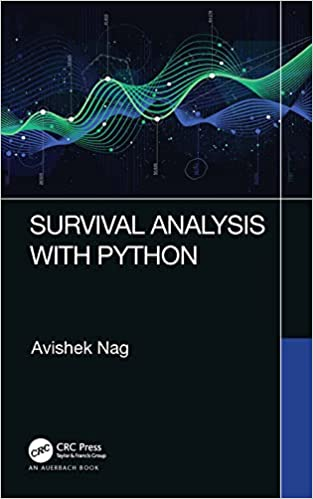Article info
• Views: 0
• Author: rukensai
• Date: 18-11-2021, 17:18
18-11-2021, 17:18

### Survival Analysis with Python

Category: E-BooksEnglish | 2021 | ISBN: ‎ 1032148268 | 94 pages | True PDF | 9.89 MB

Survival analysis uses statistics to calculate time to failure. Survival Analysis with Python takes a fresh look at this complex subject by explaining how to use the Python programming language to perform this type of analysis As the subject itself is very mathematical and full of expressions and formulations, the book provides detailed explanations and examines practical implications. The book begins with an overview of the concepts underpinning statistical survival analysis. It then delves into:

Parametric models with coverage of:
Concept of maximum likelihood estimate (MLE) of a probability distribution parameter
MLE of the survival function
Common probability distributions and their analysis
Analysis of exponential distribution as a survival function
Analysis of Weibull distribution as a survival function
Derivation of Gumbel distribution as a survival function from Weibull
Nonparametric models including:
Kaplan-Meier (KM) estimator, a derivation of expression using MLE
Fitting KM estimator with an example dataset, Python code, and plotting curves
Greenwood’s formulae and its derivation
Models with covariates explaining:
The concept of time shift and the Accelerated Life Time model (AFT)
Weibull AFT model and derivation of parameters by MLE
Proportional Hazard (PH) model
Cox-PH model
Significance of covariates
Selection of covariates

The Python lifelines library is used for coding examples. Mapping theory to practical examples featuring datasets, the book is a hands-on tutorial as well as a handy reference